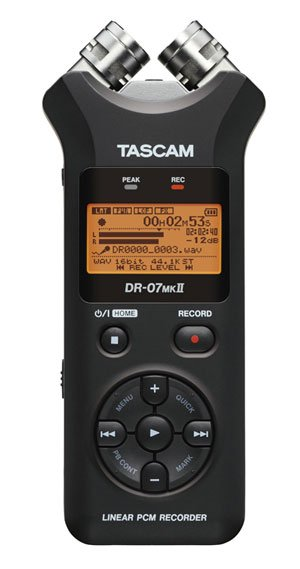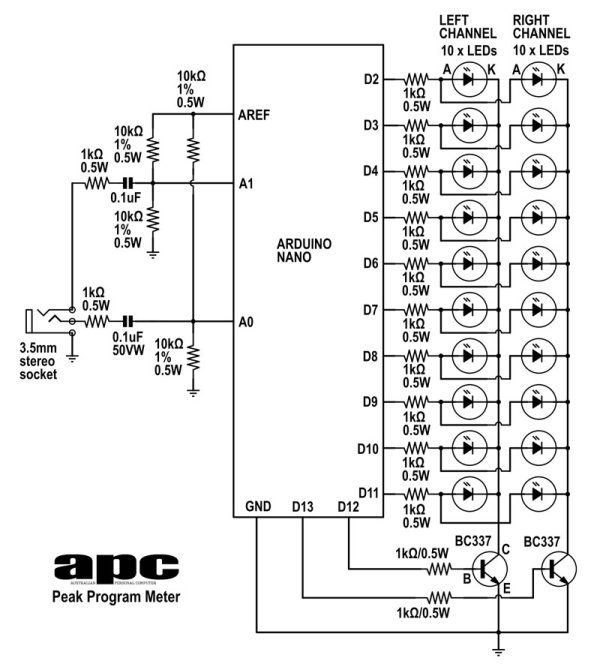# Arduino project: Stereo Peak Program Meter using arduino

Previously, we introduced the Arduino’s analog-to-digital converter (ADC) in detail, looking at successive-approximation A-D conversion and how it’s the best compromise between speed and cost. This time, we start putting some of that theory into practice by building a stereo peak-program meter. Our Peak Program Meter (pictured above) takes audio from any phone or tablet.### Modern-day VU meter

VU or ‘volume unit’ meters have been around for years as a visual aid used by professional audio engineers to gauge the voltage levels of an audio signal. Today with the prevalence of digital audio recording, a different type of meter is more commonly used that presents a linear display of a logarithmic voltage scale and it’s called a ‘peak program meter’ or PPM. When you’re recording audio with a digital recording device, the rule is never allow the signal level to exceed the maximum sample level (known as 0dBFS); otherwise, you end up with clipping distortion, which sounds like your music is playing through a gravel pit. A PPM gives you a clear indication of how ‘close to the wind’ you’re sailing.

### How it works

Digitising audio turns an analog voltage into a digital representation or ‘sample’ — the number of bits in the sample determines its precision (and helps with accuracy). It’s why 16-bit audio almost always sounds better than 8-bit audio — more bits, greater precision, better accuracy.

In the real world, the louder the sound, the larger the voltage — and when sampling that voltage, the closer to the maximum digital sample it’ll be. This maximum sample is known as ‘full scale’. Audio signals are typically measured using a logarithmic scale called decibels (dB) and our PPM measures the in-coming audio signal as a logarithmic ratio with respect to this ‘full scale’ limit. For an incoming signal that is at the maximum sample level, that ratio is said to be 0dB relative to Full Scale (FS) — and that’s how we get the ‘0dBFS’ tag.

READ  Arduino datalogging accelerometer with µ-SD storage

To work out this relative ratio of an incoming audio sample, you use the basic equation:

`dB = 20 x log10(input/FS)`

Where ‘input’ is the input signal as a digital sample level, ‘FS’ is the maximum sample level and ‘log10’ is the log function to base 10.

Let’s say the input signal level was half-FS — that would be dB = 20 x log (0.5 / 1) = 20 log (0.5) = -6.02dB. If the input was only at 10% of FS, it’d be dB = 20 log (0.1) = -20dB. With the input at 1/100th of FS, that works out to be dB = 20 log (0.01) = -40dB.

Hopefully you can see a few things from this — every time you drop half the signal level, you drop 6dB; every order-of-magnitude drop (or divide-by-10), you drop 20dB. Since the signal level is always less than full scale, the logarithmic scale always produces a negative number — the larger the absolute number, the smaller the input signal.

### Dynamic range

When you sample an audio signal, the number of bits in the ADC determines what’s called the ‘dynamic range’, the ratio between maximum and minimum samples (or loudest and softest sounds) it can capture. The 16-bit ADC in your PC’s sound card can capture 65,535 possible signal steps. Using a slight twist to our equation, we get:

`dB = 20 x log10 (ADC steps) = 20 log10 (65535) = 96.32dB`

You may have heard that audio CDs (also 16-bit sampled) have a dynamic range of 96dB — this is how they get it. However, the ADC in the Arduino’s ATMEGA328P microcontroller is only 10-bit, which gives a maximum dynamic range of 20 x log10 (1024) = 60.2dB. So from that, we know we can only cover a very tiny portion of that 16-bit range — the top 1,024 rungs in a 65,535-step ladder if you like — but represented as a logarithmic scale, we actually cover more than half of that range (54 of 96dB), which is more than enough for our PPM to do its thing.

READ  WATER LEVEL MEASUREMENT USING ARDUINO UNO R3 AND WATER SENSORS

### Making our display

As we said at the top, a PPM is a linear display of a logarithmic scale. We’ve covered the log scale; now let’s look at the linear display. What we want to do is produce a stereo (right- and left-channel) bargraph-style display, so the first thing we need to do is look at the digital I/O ports we have to play with.

For this project, we’re using the Arduino Nano, a compact, ‘breadboard-able’ version of the Arduino Uno R3 using the same ATMEGA328P microcontroller.The PPM’s linear scale is designed to make it easy to follow since each LED represents a fixed decibel step, however, most PPMs built from discrete components only manage an approximate logarithmic scale or ‘decibel linearity’ because it’s actually quite hard to do. Our meter gets around that problem but also has perfect decibel linearity, thanks to the ‘mathemagic’ we do inside the Arduino. And as a little something extra, you can set the decibel-per-LED scale in the Arduino sketch (code).

For more detail: Arduino project: Stereo Peak Program Meter

#### This Post / Project can also be found using search terms:

• arduino stereo vu meter
• Arduino stereo vu
• peak program meter
• peak program meter analog